# User:IssaRice/Computability and logic/Diagonalization out of a class

Let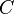$C$ be some class of total functions. If we are given an enumeration of$C$ such as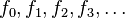$f_0, f_1, f_2, f_3, \ldots$ then we can define a new total function$g(x) := f_x(x) + 1$. Now given any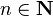$n \in \mathbf N$, we have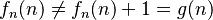$f_n(n) \ne f_n(n) + 1 = g(n)$. Thus,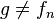$g\ne f_n$ for every$n\in \mathbf N$, so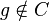$g\notin C$.